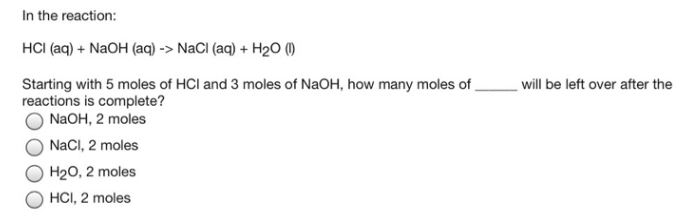# Problem: In the reaction: HCI (aq) + NaOH (aq) → NaCl (aq) + H 2O (I)Starting with 5 moles of HCI and 3 moles of NaOH, how many moles of ___ will be left over after the reactions is complete?a. NaOH, 2 molesb. NaCl, 2 molesc. H2O, 2 molesd. HCl, 2 moles

###### FREE Expert Solution###### Problem Details

In the reaction:

HCI (aq) + NaOH (aq) → NaCl (aq) + H 2O (I)

Starting with 5 moles of HCI and 3 moles of NaOH, how many moles of ___ will be left over after the reactions is complete?

a. NaOH, 2 moles

b. NaCl, 2 moles

c. H2O, 2 moles

d. HCl, 2 moles